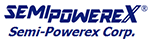HOME > >
IGBT Modules
• SPM
• 100
• GB
• 601
• S1
 Ⅰ Trade Mark for IGBT Modules Ⅱ Current : I с @ T с =80℃ Ⅲ Topology : GA = Single GAL(R) = Chopper GB = Half Bridge (2-Pack) GD = Full Bridge (4-Pack) Ⅳ Blocking Voltage + IGBT type601 = 600 V NPT1201 = 1200 V SPT+ 1202 = 1200 V NPT Ⅴ Package : S1 = 34mmS3 = 62mmS4 = 38mmS7 = SOT-227
Diode Modules
• SPD
• 100
• CC
• 60
• S1
 Ⅰ Trade Mark for Diode Modules Ⅱ Current : I с @ T с =80℃ Ⅲ Topology : CC = Common CathodeCA = Common AnodeSC = Side Common CathodeSA = Side Common AnodeKA = Cathode Anode Ⅳ Blocking Voltage 40 = 400 V 60 = 600 V 120 = 1200 V Ⅴ Package : SH = 27mmS1 = 34mmST = 40mmS7 = SOT-227